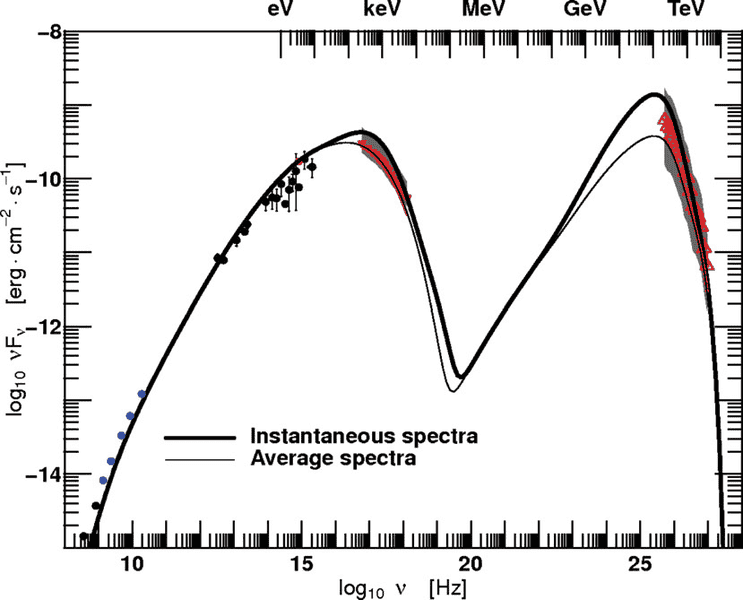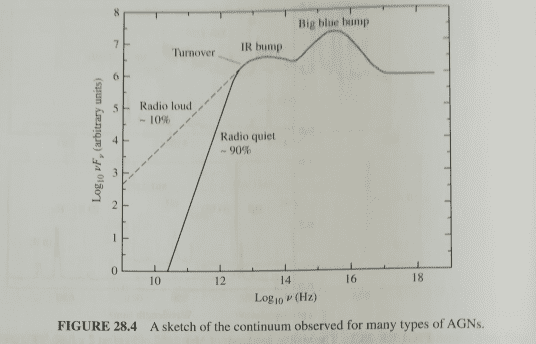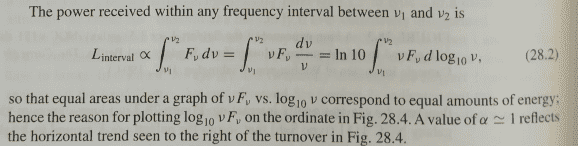# Astrophysics simple question about luminosity

• I
• Astroiaco
In summary, frequency is used to identify nuclei, while luminosity will tell you how much. The product νLν is used to reduce the size of the graph, because it is expected to account for the variations across the whole electromagnetic range.f

#### Astroiaco

Why in Astrophysics it is used νLν (where ν is the frequency and Lν is the luminosity at that frequency) in the emission spectrum of an accretion disk?

Frequency is used to identify nuclei, while luminosity will tell you how much.

Ok, but why often in the plot of luminosity (where there are ν in abscissa and ν*Lν in ordinate) there is the product between ν and Lν and not only Lν? I don't know if i explained myself better.

Accretion disk are of different scales, depending on the mass of the black hole. But guessing from your profile pic and the similarity of the field I assume you are talking about the supermassive black hole accreting galactic matter; more precisely Active Galactic Nuclei(AGNs).

but why often in the plot of luminosity (where there are ν in abscissa and ν*Lν in ordinate) there is the product between ν and Lν and not only Lν?
Here's why I think it is so: to reduce the graph size!

There's something you missed, sometimes the graph is plotted logarithmically, otherwise in powers of 10. This is because the Spectrum is expected to account for the variations across the whole electromagnetic range, and hence the graph would be too big if plotted in normal units.A similar reasoning goes for plotting the product νLν. In log units this is easier to see. Notice that log(νLν)=logν+logLν. Now look at the above graph, a simple subtraction will tell you that the y-axis of the graph scales from -24 to -35 if only logLν is plotted.

Another reason might be by convention. Usually AGNs have a typical double hump(the shapes vary for different sources) structure when plotted as a product. In field they usually fit the different emission mechanics to this double hump structure; not that you can't do this for just luminosity, it's just what they do.

PS: Why I think this can't be from concrete theoretical calculation? Well, it doesn't matter if you plot luminosity or the product of luminosity and frequency, since the only quantity with nontrivial variations is the luminosity. Besides there are options in plotting program to plot ν2Lν.

#### Attachments

•Astroiaco
Why in Astrophysics it is used νLν (where ν is the frequency and Lν is the luminosity at that frequency) in the emission spectrum of an accretion disk?

Could you pls provide a reference source? I suspect that ##L_{\nu}## would be a spectral luminosity, i.e. luminosity per an unit frequency interval, measured in units ##(W/Hz)##. By multiplying this value and a specific frequency interval ##\nu##, you should get the total luminosity in Watts over this specific interval.

•Astroiaco
Could you pls provide a reference source? I suspect that ##L_{\nu}## would be a spectral luminosity, i.e. luminosity per an unit frequency interval, measured in units ##(W/Hz)##. By multiplying this value and a specific frequency interval ##\nu##, you should get the total luminosity in Watts over this specific interval.
I'm studying the AGNs for my undergraduate thesis, so i have many reference sources, but for example you can find this type of writing on: Dan Maoz "Astrophysics in a Nutshell".

Yes, ##L_{\nu}## is measured in units ##(W/Hz)##.

Accretion disk are of different scales, depending on the mass of the black hole. But guessing from your profile pic and the similarity of the field I assume you are talking about the supermassive black hole accreting galactic matter; more precisely Active Galactic Nuclei(AGNs).

Here's why I think it is so: to reduce the graph size!

There's something you missed, sometimes the graph is plotted logarithmically, otherwise in powers of 10. This is because the Spectrum is expected to account for the variations across the whole electromagnetic range, and hence the graph would be too big if plotted in normal units.
View attachment 230147

A similar reasoning goes for plotting the product νLν. In log units this is easier to see. Notice that log(νLν)=logν+logLν. Now look at the above graph, a simple subtraction will tell you that the y-axis of the graph scales from -24 to -35 if only logLν is plotted.

Another reason might be by convention. Usually AGNs have a typical double hump(the shapes vary for different sources) structure when plotted as a product. In field they usually fit the different emission mechanics to this double hump structure; not that you can't do this for just luminosity, it's just what they do.

PS: Why I think this can't be from concrete theoretical calculation? Well, it doesn't matter if you plot luminosity or the product of luminosity and frequency, since the only quantity with nontrivial variations is the luminosity. Besides there are options in plotting program to plot ν2Lν.

Thank you very much!

I'm studying the AGNs for my undergraduate thesis, so i have many reference sources, but for example you can find this type of writing on: Dan Maoz "Astrophysics in a Nutshell".

Yes, ##L_{\nu}## is measured in units ##(W/Hz)##.

Ah good, I think I found quite a nice explanation in Carroll&Ostlies: "An Introduction to Modern Astrophysics", two snapshots below. If I got it right, when you construct graph like this, the area under the curve of an interval will be proportional to the amount of energy received in that interval (i.e. "equal areas under the graph correspond to equal amounts of energy").#### Attachments

•Astroiaco